### Third Law of Thermodynamics

The third law of thermodynamics is often also called NERNST's2.11 heat theorem. It is related to the quantum mechanical regime of a given system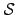. For the special case that the temperature reaches very low values in the zero Kelvin regime, the entropy becomes a constant value due to the finite states. The minimum number of possible microstates is one if a system is considered which consists of vacuum only, the corresponding minimum entropy is zero. Hence, the logarithmic value becomes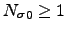and the minimum of the entropy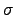is determined as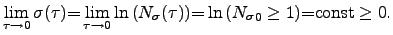(2.41)

Therefore, the values of the entropyat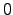K is expected to be very small, except for some materials where a frozen internal disorder can be observed at temperatures aboveK, e.g. in glasses and ceramics .

Most of the stationary heating phenomena can be described by the fundamental laws of thermodynamic. These equations can be used for the local thermal equilibrium and the local quasi thermal equilibrium if the system is not too far away from the local thermal equilibrium. The transient behavior of a system becomes more important when the investigated systems have raising numbers of uncertainties of their internal state variables. Therefore, the global energy of the system is being minimized according to the second fundamental law of thermodynamics.

Analogously to the observed behavior of fluids and gases, heat transport can be treated as a kind of energy transport, for instance within the electron gas, as it is shown in the following sections.

Stefan Holzer 2007-11-19